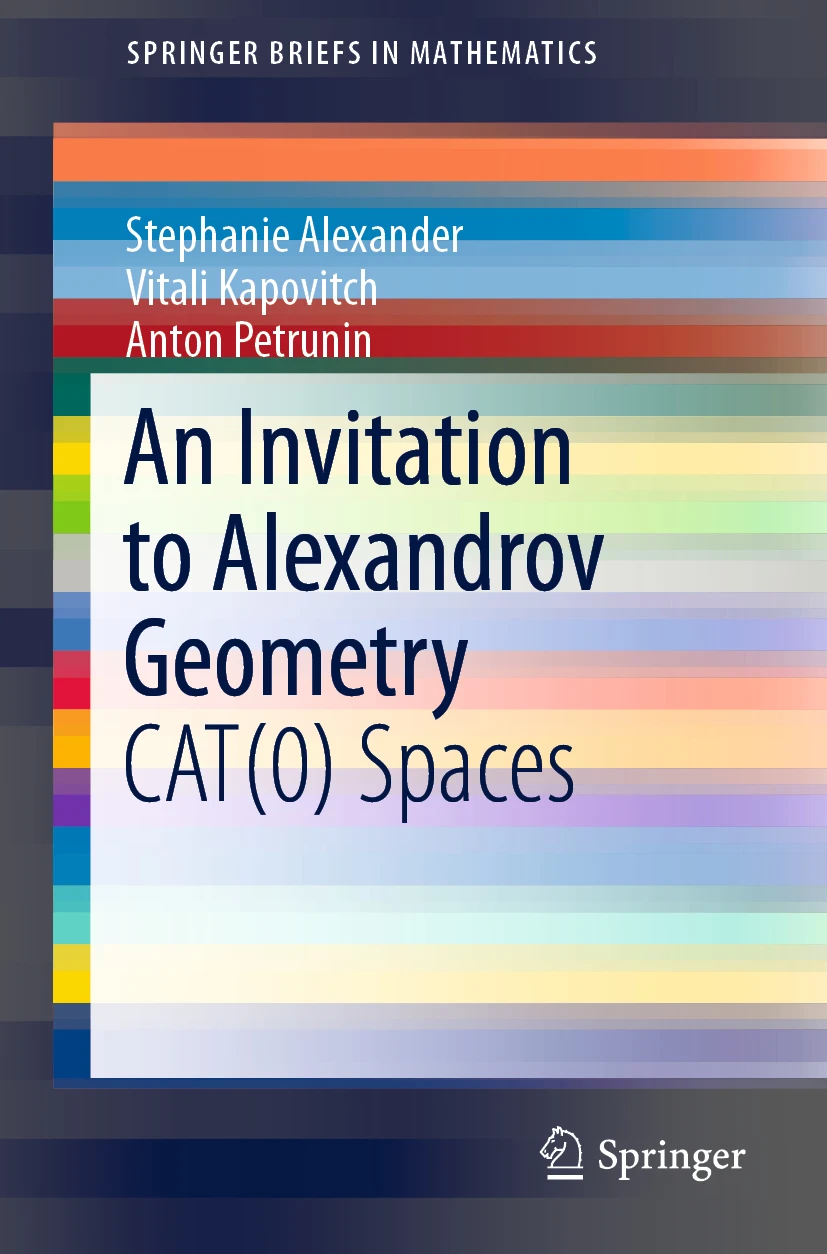## An invitation to Alexandrov geometry: CAT(0) spacesThe source files can be downloaded from the project page on GitHub; the final publication is available at Springer via DOI: 10.1007/978-3-030-05312-3. An older (CC BY-SA)-version is available at arXiv:1701.03483.

The idea is to demonstrate the beauty and power of Alexandrov geometry by reaching interesting applications and theorems with a minimum of preparation. These notes arise as an offshoot of the book on Alexandrov geometry we have been writing for a number of years. The notes were shaped in a number of lectures given by the third author to undergraduate students at different occasions at the MASS program at Penn State University and the Summer School “Algebra and Geometry” in Yaroslavl.

Chapter 1 includes the necessary preliminaries.

In Chapter 2, we discuss the Reshetnyak gluing theorem and apply it to a problem in billiards which was solved by Dmitri Burago, Serge Ferleger and Alexey Kononenko.

In Chapter 3, we discuss the Hadamard–Cartan globalization theorem, and apply it to the construction of exotic aspherical manifolds introduced by Michael Davis.

In Chapter 4, we discuss examples of Alexandrov spaces with curvature bounded above. This chapter is based largely on work of Samuel Shefel on nonsmooth saddle surfaces.

## Misprints in the published version

• The numbers in some citations are shifted by 1; at least one citation is missing.
• page 34--35, the patchwork along a curve (3.2.2) has to be formulated for local geodesics only; minor changes in the proof are required.
• page 44, line $$-4$$. The following
"By the globalization theorem (3.3.1), any proper length CAT(0) space is contractible. Therefore all proper..."
has to be changed to
"By 2.2.6, any proper length CAT(0) space is contractible. Therefore, by the globalization theorem (3.3.1), all proper..."
• page 53, line 15: $$\hat{\varphi^{-1}}(W)$$ $$\to$$ $$\hat\varphi^{-1}(W)$$